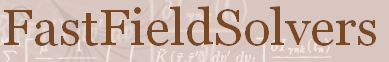FastFieldSolvers Forum
Home | Profile | Register | Active Topics | Members | Search | FAQ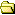All Forums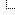FastFieldSolvers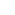FastHenry2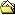Segs dont seem parallel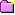New Topic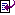Reply to Topic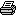Printer Friendly
Author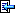Topic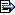Spain
3 Posts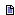Posted - Mar 24 2019 :  16:19:44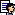Hello.I'm having trouble simulating the coupling between two multilayer flat inductors turned 45�.The solver keeps spaming the message:uh oh, segs don't seem parallel -0.000585789This doesn't happen when i simulate them without turning them around.Could this be a resolution problem?This is the model file:* FastHenry2 File Automatically Generated.... JCCopyrights 2019.Units M.Default z=0 sigma=5.8e4 w=1 h=1 nhinc=2 nwinc=2*Coil 1 Nodes:N1 x=-0.015 y=0.015 z=0.0048N2 x=0.015 y=0.015 z=0.0048N3 x=0.015 y=-0.015 z=0.0048N4 x=-0.015 y=-0.015 z=0.0048N5 x=-0.015 y=0.01234 z=0.0048N6 x=-0.01234 y=0.01234 z=0.0048N7 x=0.01234 y=0.01234 z=0.0048N8 x=0.01234 y=-0.01234 z=0.0048N9 x=-0.01234 y=-0.01234 z=0.0048N10 x=-0.01234 y=0.00968 z=0.0048N11 x=-0.00968 y=0.00968 z=0.0048N12 x=0.00968 y=0.00968 z=0.0048N13 x=0.00968 y=-0.00968 z=0.0048N14 x=-0.00968 y=-0.00968 z=0.0048N15 x=-0.00968 y=0.00702 z=0.0048N16 x=-0.00702 y=0.00702 z=0.0048N17 x=0.00702 y=0.00702 z=0.0048N18 x=0.00702 y=-0.00702 z=0.0048N19 x=-0.00702 y=-0.00702 z=0.0048N20 x=-0.00702 y=0.00436 z=0.0048N21 x=-0.00436 y=0.00436 z=0.0048N22 x=0.00436 y=0.00436 z=0.0048N23 x=0.00436 y=-0.00436 z=0.0048N24 x=-0.00436 y=-0.00436 z=0.0048N25 x=-0.00436 y=0.0017 z=0.0048N26 x=-0.0017 y=0.0017 z=0.0048N27 x=0.0017 y=0.0017 z=0.0048N28 x=0.0017 y=-0.0017 z=0.0048N29 x=-0.0017 y=-0.0017 z=0.0048N30 x=-0.0017 y=-0.00096 z=0.0048N31 x=-0.0017 y=-0.00096 z=0.0032N32 x=-0.0017 y=0.0017 z=0.0032N33 x=0.0017 y=0.0017 z=0.0032N34 x=0.0017 y=-0.0017 z=0.0032N35 x=-0.0017 y=-0.0017 z=0.0032N36 x=-0.00436 y=-0.0017 z=0.0032N37 x=-0.00436 y=0.00436 z=0.0032N38 x=0.00436 y=0.00436 z=0.0032N39 x=0.00436 y=-0.00436 z=0.0032N40 x=-0.00436 y=-0.00436 z=0.0032N41 x=-0.00702 y=-0.00436 z=0.0032N42 x=-0.00702 y=0.00702 z=0.0032N43 x=0.00702 y=0.00702 z=0.0032N44 x=0.00702 y=-0.00702 z=0.0032N45 x=-0.00702 y=-0.00702 z=0.0032N46 x=-0.00968 y=-0.00702 z=0.0032N47 x=-0.00968 y=0.00968 z=0.0032N48 x=0.00968 y=0.00968 z=0.0032N49 x=0.00968 y=-0.00968 z=0.0032N50 x=-0.00968 y=-0.00968 z=0.0032N51 x=-0.01234 y=-0.00968 z=0.0032N52 x=-0.01234 y=0.01234 z=0.0032N53 x=0.01234 y=0.01234 z=0.0032N54 x=0.01234 y=-0.01234 z=0.0032N55 x=-0.01234 y=-0.01234 z=0.0032N56 x=-0.015 y=-0.01234 z=0.0032N57 x=-0.015 y=0.015 z=0.0032N58 x=0.015 y=0.015 z=0.0032N59 x=0.015 y=-0.015 z=0.0032N60 x=-0.015 y=-0.015 z=0.0032N61 x=-0.01766 y=-0.015 z=0.0032N62 x=-0.01766 y=0.015 z=0.0032N63 x=-0.01766 y=0.015 z=0.0016N64 x=-0.015 y=0.015 z=0.0016N65 x=0.015 y=0.015 z=0.0016N66 x=0.015 y=-0.015 z=0.0016N67 x=-0.015 y=-0.015 z=0.0016N68 x=-0.015 y=0.01234 z=0.0016N69 x=-0.01234 y=0.01234 z=0.0016N70 x=0.01234 y=0.01234 z=0.0016N71 x=0.01234 y=-0.01234 z=0.0016N72 x=-0.01234 y=-0.01234 z=0.0016N73 x=-0.01234 y=0.00968 z=0.0016N74 x=-0.00968 y=0.00968 z=0.0016N75 x=0.00968 y=0.00968 z=0.0016N76 x=0.00968 y=-0.00968 z=0.0016N77 x=-0.00968 y=-0.00968 z=0.0016N78 x=-0.00968 y=0.00702 z=0.0016N79 x=-0.00702 y=0.00702 z=0.0016N80 x=0.00702 y=0.00702 z=0.0016N81 x=0.00702 y=-0.00702 z=0.0016N82 x=-0.00702 y=-0.00702 z=0.0016N83 x=-0.00702 y=0.00436 z=0.0016N84 x=-0.00436 y=0.00436 z=0.0016N85 x=0.00436 y=0.00436 z=0.0016N86 x=0.00436 y=-0.00436 z=0.0016N87 x=-0.00436 y=-0.00436 z=0.0016N88 x=-0.00436 y=0.0017 z=0.0016N89 x=-0.0017 y=0.0017 z=0.0016N90 x=0.0017 y=0.0017 z=0.0016N91 x=0.0017 y=-0.0017 z=0.0016N92 x=-0.0017 y=-0.0017 z=0.0016N93 x=-0.0017 y=-0.00096 z=0.0016N94 x=-0.0017 y=-0.00096 z=0N95 x=-0.0017 y=0.0017 z=0N96 x=0.0017 y=0.0017 z=0N97 x=0.0017 y=-0.0017 z=0N98 x=-0.0017 y=-0.0017 z=0N99 x=-0.00436 y=-0.0017 z=0N100 x=-0.00436 y=0.00436 z=-8.67362e-19N101 x=0.00436 y=0.00436 z=-8.67362e-19N102 x=0.00436 y=-0.00436 z=8.67362e-19N103 x=-0.00436 y=-0.00436 z=8.67362e-19N104 x=-0.00702 y=-0.00436 z=8.67362e-19N105 x=-0.00702 y=0.00702 z=-8.67362e-19N106 x=0.00702 y=0.00702 z=-8.67362e-19N107 x=0.00702 y=-0.00702 z=8.67362e-19N108 x=-0.00702 y=-0.00702 z=8.67362e-19N109 x=-0.00968 y=-0.00702 z=8.67362e-19N110 x=-0.00968 y=0.00968 z=-8.67362e-19N111 x=0.00968 y=0.00968 z=-8.67362e-19N112 x=0.00968 y=-0.00968 z=8.67362e-19N113 x=-0.00968 y=-0.00968 z=8.67362e-19N114 x=-0.01234 y=-0.00968 z=8.67362e-19N115 x=-0.01234 y=0.01234 z=-1.73472e-18N116 x=0.01234 y=0.01234 z=-1.73472e-18N117 x=0.01234 y=-0.01234 z=1.73472e-18N118 x=-0.01234 y=-0.01234 z=1.73472e-18N119 x=-0.015 y=-0.01234 z=1.73472e-18N120 x=-0.015 y=0.015 z=-1.73472e-18N121 x=0.015 y=0.015 z=-1.73472e-18N122 x=0.015 y=-0.015 z=1.73472e-18N123 x=-0.015 y=-0.015 z=1.73472e-18N124 x=-0.01766 y=-0.015 z=1.73472e-18N125 x=-0.01766 y=0.015 z=1.73472e-18*Coil 1 Segments:E1 N1 N2 w = 0.00133 h = 0.00133 sigma = 5.96e+07 nhinc = 4 nwinc = 4 rh = 10 rw = 10E2 N2 N3 w = 0.00133 h = 0.00133 sigma = 5.96e+07 nhinc = 4 nwinc = 4 rh = 10 rw = 10E3 N3 N4 w = 0.00133 h = 0.00133 sigma = 5.96e+07 nhinc = 4 nwinc = 4 rh = 10 rw = 10E4 N4 N5 w = 0.00133 h = 0.00133 sigma = 5.96e+07 nhinc = 4 nwinc = 4 rh = 10 rw = 10E5 N5 N6 w = 0.00133 h = 0.00133 sigma = 5.96e+07 nhinc = 4 nwinc = 4 rh = 10 rw = 10E6 N6 N7 w = 0.00133 h = 0.00133 sigma = 5.96e+07 nhinc = 4 nwinc = 4 rh = 10 rw = 10E7 N7 N8 w = 0.00133 h = 0.00133 sigma = 5.96e+07 nhinc = 4 nwinc = 4 rh = 10 rw = 10E8 N8 N9 w = 0.00133 h = 0.00133 sigma = 5.96e+07 nhinc = 4 nwinc = 4 rh = 10 rw = 10E9 N9 N10 w = 0.00133 h = 0.00133 sigma = 5.96e+07 nhinc = 4 nwinc = 4 rh = 10 rw = 10E10 N10 N11 w = 0.00133 h = 0.00133 sigma = 5.96e+07 nhinc = 4 nwinc = 4 rh = 10 rw = 10E11 N11 N12 w = 0.00133 h = 0.00133 sigma = 5.96e+07 nhinc = 4 nwinc = 4 rh = 10 rw = 10E12 N12 N13 w = 0.00133 h = 0.00133 sigma = 5.96e+07 nhinc = 4 nwinc = 4 rh = 10 rw = 10E13 N13 N14 w = 0.00133 h = 0.00133 sigma = 5.96e+07 nhinc = 4 nwinc = 4 rh = 10 rw = 10E14 N14 N15 w = 0.00133 h = 0.00133 sigma = 5.96e+07 nhinc = 4 nwinc = 4 rh = 10 rw = 10E15 N15 N16 w = 0.00133 h = 0.00133 sigma = 5.96e+07 nhinc = 4 nwinc = 4 rh = 10 rw = 10E16 N16 N17 w = 0.00133 h = 0.00133 sigma = 5.96e+07 nhinc = 4 nwinc = 4 rh = 10 rw = 10E17 N17 N18 w = 0.00133 h = 0.00133 sigma = 5.96e+07 nhinc = 4 nwinc = 4 rh = 10 rw = 10E18 N18 N19 w = 0.00133 h = 0.00133 sigma = 5.96e+07 nhinc = 4 nwinc = 4 rh = 10 rw = 10E19 N19 N20 w = 0.00133 h = 0.00133 sigma = 5.96e+07 nhinc = 4 nwinc = 4 rh = 10 rw = 10E20 N20 N21 w = 0.00133 h = 0.00133 sigma = 5.96e+07 nhinc = 4 nwinc = 4 rh = 10 rw = 10E21 N21 N22 w = 0.00133 h = 0.00133 sigma = 5.96e+07 nhinc = 4 nwinc = 4 rh = 10 rw = 10E22 N22 N23 w = 0.00133 h = 0.00133 sigma = 5.96e+07 nhinc = 4 nwinc = 4 rh = 10 rw = 10E23 N23 N24 w = 0.00133 h = 0.00133 sigma = 5.96e+07 nhinc = 4 nwinc = 4 rh = 10 rw = 10E24 N24 N25 w = 0.00133 h = 0.00133 sigma = 5.96e+07 nhinc = 4 nwinc = 4 rh = 10 rw = 10E25 N25 N26 w = 0.00133 h = 0.00133 sigma = 5.96e+07 nhinc = 4 nwinc = 4 rh = 10 rw = 10E26 N26 N27 w = 0.00133 h = 0.00133 sigma = 5.96e+07 nhinc = 4 nwinc = 4 rh = 10 rw = 10E27 N27 N28 w = 0.00133 h = 0.00133 sigma = 5.96e+07 nhinc = 4 nwinc = 4 rh = 10 rw = 10E28 N28 N29 w = 0.00133 h = 0.00133 sigma = 5.96e+07 nhinc = 4 nwinc = 4 rh = 10 rw = 10E29 N29 N30 w = 0.00133 h = 0.00133 sigma = 5.96e+07 nhinc = 4 nwinc = 4 rh = 10 rw = 10E30 N30 N31 w = 0.00133 h = 0.00133 sigma = 5.96e+07 nhinc = 4 nwinc = 4 rh = 10 rw = 10E31 N31 N32 w = 0.00133 h = 0.00133 sigma = 5.96e+07 nhinc = 4 nwinc = 4 rh = 10 rw = 10E32 N32 N33 w = 0.00133 h = 0.00133 sigma = 5.96e+07 nhinc = 4 nwinc = 4 rh = 10 rw = 10E33 N33 N34 w = 0.00133 h = 0.00133 sigma = 5.96e+07 nhinc = 4 nwinc = 4 rh = 10 rw = 10E34 N34 N35 w = 0.00133 h = 0.00133 sigma = 5.96e+07 nhinc = 4 nwinc = 4 rh = 10 rw = 10E35 N35 N36 w = 0.00133 h = 0.00133 sigma = 5.96e+07 nhinc = 4 nwinc = 4 rh = 10 rw = 10E36 N36 N37 w = 0.00133 h = 0.00133 sigma = 5.96e+07 nhinc = 4 nwinc = 4 rh = 10 rw = 10E37 N37 N38 w = 0.00133 h = 0.00133 sigma = 5.96e+07 nhinc = 4 nwinc = 4 rh = 10 rw = 10E38 N38 N39 w = 0.00133 h = 0.00133 sigma = 5.96e+07 nhinc = 4 nwinc = 4 rh = 10 rw = 10E39 N39 N40 w = 0.00133 h = 0.00133 sigma = 5.96e+07 nhinc = 4 nwinc = 4 rh = 10 rw = 10E40 N40 N41 w = 0.00133 h = 0.00133 sigma = 5.96e+07 nhinc = 4 nwinc = 4 rh = 10 rw = 10E41 N41 N42 w = 0.00133 h = 0.00133 sigma = 5.96e+07 nhinc = 4 nwinc = 4 rh = 10 rw = 10E42 N42 N43 w = 0.00133 h = 0.00133 sigma = 5.96e+07 nhinc = 4 nwinc = 4 rh = 10 rw = 10E43 N43 N44 w = 0.00133 h = 0.00133 sigma = 5.96e+07 nhinc = 4 nwinc = 4 rh = 10 rw = 10E44 N44 N45 w = 0.00133 h = 0.00133 sigma = 5.96e+07 nhinc = 4 nwinc = 4 rh = 10 rw = 10E45 N45 N46 w = 0.00133 h = 0.00133 sigma = 5.96e+07 nhinc = 4 nwinc = 4 rh = 10 rw = 10E46 N46 N47 w = 0.00133 h = 0.00133 sigma = 5.96e+07 nhinc = 4 nwinc = 4 rh = 10 rw = 10E47 N47 N48 w = 0.00133 h = 0.00133 sigma = 5.96e+07 nhinc = 4 nwinc = 4 rh = 10 rw = 10E48 N48 N49 w = 0.00133 h = 0.00133 sigma = 5.96e+07 nhinc = 4 nwinc = 4 rh = 10 rw = 10E49 N49 N50 w = 0.00133 h = 0.00133 sigma = 5.96e+07 nhinc = 4 nwinc = 4 rh = 10 rw = 10E50 N50 N51 w = 0.00133 h = 0.00133 sigma = 5.96e+07 nhinc = 4 nwinc = 4 rh = 10 rw = 10E51 N51 N52 w = 0.00133 h = 0.00133 sigma = 5.96e+07 nhinc = 4 nwinc = 4 rh = 10 rw = 10E52 N52 N53 w = 0.00133 h = 0.00133 sigma = 5.96e+07 nhinc = 4 nwinc = 4 rh = 10 rw = 10E53 N53 N54 w = 0.00133 h = 0.00133 sigma = 5.96e+07 nhinc = 4 nwinc = 4 rh = 10 rw = 10E54 N54 N55 w = 0.00133 h = 0.00133 sigma = 5.96e+07 nhinc = 4 nwinc = 4 rh = 10 rw = 10E55 N55 N56 w = 0.00133 h = 0.00133 sigma = 5.96e+07 nhinc = 4 nwinc = 4 rh = 10 rw = 10E56 N56 N57 w = 0.00133 h = 0.00133 sigma = 5.96e+07 nhinc = 4 nwinc = 4 rh = 10 rw = 10E57 N57 N58 w = 0.00133 h = 0.00133 sigma = 5.96e+07 nhinc = 4 nwinc = 4 rh = 10 rw = 10E58 N58 N59 w = 0.00133 h = 0.00133 sigma = 5.96e+07 nhinc = 4 nwinc = 4 rh = 10 rw = 10E59 N59 N60 w = 0.00133 h = 0.00133 sigma = 5.96e+07 nhinc = 4 nwinc = 4 rh = 10 rw = 10E60 N60 N61 w = 0.00133 h = 0.00133 sigma = 5.96e+07 nhinc = 4 nwinc = 4 rh = 10 rw = 10E61 N61 N62 w = 0.00133 h = 0.00133 sigma = 5.96e+07 nhinc = 4 nwinc = 4 rh = 10 rw = 10E62 N62 N63 w = 0.00133 h = 0.00133 sigma = 5.96e+07 nhinc = 4 nwinc = 4 rh = 10 rw = 10E63 N63 N64 w = 0.00133 h = 0.00133 sigma = 5.96e+07 nhinc = 4 nwinc = 4 rh = 10 rw = 10E64 N64 N65 w = 0.00133 h = 0.00133 sigma = 5.96e+07 nhinc = 4 nwinc = 4 rh = 10 rw = 10E65 N65 N66 w = 0.00133 h = 0.00133 sigma = 5.96e+07 nhinc = 4 nwinc = 4 rh = 10 rw = 10E66 N66 N67 w = 0.00133 h = 0.00133 sigma = 5.96e+07 nhinc = 4 nwinc = 4 rh = 10 rw = 10E67 N67 N68 w = 0.00133 h = 0.00133 sigma = 5.96e+07 nhinc = 4 nwinc = 4 rh = 10 rw = 10E68 N68 N69 w = 0.00133 h = 0.00133 sigma = 5.96e+07 nhinc = 4 nwinc = 4 rh = 10 rw = 10E69 N69 N70 w = 0.00133 h = 0.00133 sigma = 5.96e+07 nhinc = 4 nwinc = 4 rh = 10 rw = 10E70 N70 N71 w = 0.00133 h = 0.00133 sigma = 5.96e+07 nhinc = 4 nwinc = 4 rh = 10 rw = 10E71 N71 N72 w = 0.00133 h = 0.00133 sigma = 5.96e+07 nhinc = 4 nwinc = 4 rh = 10 rw = 10E72 N72 N73 w = 0.00133 h = 0.00133 sigma = 5.96e+07 nhinc = 4 nwinc = 4 rh = 10 rw = 10E73 N73 N74 w = 0.00133 h = 0.00133 sigma = 5.96e+07 nhinc = 4 nwinc = 4 rh = 10 rw = 10E74 N74 N75 w = 0.00133 h = 0.00133 sigma = 5.96e+07 nhinc = 4 nwinc = 4 rh = 10 rw = 10E75 N75 N76 w = 0.00133 h = 0.00133 sigma = 5.96e+07 nhinc = 4 nwinc = 4 rh = 10 rw = 10E76 N76 N77 w = 0.00133 h = 0.00133 sigma = 5.96e+07 nhinc = 4 nwinc = 4 rh = 10 rw = 10E77 N77 N78 w = 0.00133 h = 0.00133 sigma = 5.96e+07 nhinc = 4 nwinc = 4 rh = 10 rw = 10E78 N78 N79 w = 0.00133 h = 0.00133 sigma = 5.96e+07 nhinc = 4 nwinc = 4 rh = 10 rw = 10E79 N79 N80 w = 0.00133 h = 0.00133 sigma = 5.96e+07 nhinc = 4 nwinc = 4 rh = 10 rw = 10E80 N80 N81 w = 0.00133 h = 0.00133 sigma = 5.96e+07 nhinc = 4 nwinc = 4 rh = 10 rw = 10E81 N81 N82 w = 0.00133 h = 0.00133 sigma = 5.96e+07 nhinc = 4 nwinc = 4 rh = 10 rw = 10E82 N82 N83 w = 0.00133 h = 0.00133 sigma = 5.96e+07 nhinc = 4 nwinc = 4 rh = 10 rw = 10E83 N83 N84 w = 0.00133 h = 0.00133 sigma = 5.96e+07 nhinc = 4 nwinc = 4 rh = 10 rw = 10E84 N84 N85 w = 0.00133 h = 0.00133 sigma = 5.96e+07 nhinc = 4 nwinc = 4 rh = 10 rw = 10E85 N85 N86 w = 0.00133 h = 0.00133 sigma = 5.96e+07 nhinc = 4 nwinc = 4 rh = 10 rw = 10E86 N86 N87 w = 0.00133 h = 0.00133 sigma = 5.96e+07 nhinc = 4 nwinc = 4 rh = 10 rw = 10E87 N87 N88 w = 0.00133 h = 0.00133 sigma = 5.96e+07 nhinc = 4 nwinc = 4 rh = 10 rw = 10E88 N88 N89 w = 0.00133 h = 0.00133 sigma = 5.96e+07 nhinc = 4 nwinc = 4 rh = 10 rw = 10E89 N89 N90 w = 0.00133 h = 0.00133 sigma = 5.96e+07 nhinc = 4 nwinc = 4 rh = 10 rw = 10E90 N90 N91 w = 0.00133 h = 0.00133 sigma = 5.96e+07 nhinc = 4 nwinc = 4 rh = 10 rw = 10E91 N91 N92 w = 0.00133 h = 0.00133 sigma = 5.96e+07 nhinc = 4 nwinc = 4 rh = 10 rw = 10E92 N92 N93 w = 0.00133 h = 0.00133 sigma = 5.96e+07 nhinc = 4 nwinc = 4 rh = 10 rw = 10E93 N93 N94 w = 0.00133 h = 0.00133 sigma = 5.96e+07 nhinc = 4 nwinc = 4 rh = 10 rw = 10E94 N94 N95 w = 0.00133 h = 0.00133 sigma = 5.96e+07 nhinc = 4 nwinc = 4 rh = 10 rw = 10E95 N95 N96 w = 0.00133 h = 0.00133 sigma = 5.96e+07 nhinc = 4 nwinc = 4 rh = 10 rw = 10E96 N96 N97 w = 0.00133 h = 0.00133 sigma = 5.96e+07 nhinc = 4 nwinc = 4 rh = 10 rw = 10E97 N97 N98 w = 0.00133 h = 0.00133 sigma = 5.96e+07 nhinc = 4 nwinc = 4 rh = 10 rw = 10E98 N98 N99 w = 0.00133 h = 0.00133 sigma = 5.96e+07 nhinc = 4 nwinc = 4 rh = 10 rw = 10E99 N99 N100 w = 0.00133 h = 0.00133 sigma = 5.96e+07 nhinc = 4 nwinc = 4 rh = 10 rw = 10E100 N100 N101 w = 0.00133 h = 0.00133 sigma = 5.96e+07 nhinc = 4 nwinc = 4 rh = 10 rw = 10E101 N101 N102 w = 0.00133 h = 0.00133 sigma = 5.96e+07 nhinc = 4 nwinc = 4 rh = 10 rw = 10E102 N102 N103 w = 0.00133 h = 0.00133 sigma = 5.96e+07 nhinc = 4 nwinc = 4 rh = 10 rw = 10E103 N103 N104 w = 0.00133 h = 0.00133 sigma = 5.96e+07 nhinc = 4 nwinc = 4 rh = 10 rw = 10E104 N104 N105 w = 0.00133 h = 0.00133 sigma = 5.96e+07 nhinc = 4 nwinc = 4 rh = 10 rw = 10E105 N105 N106 w = 0.00133 h = 0.00133 sigma = 5.96e+07 nhinc = 4 nwinc = 4 rh = 10 rw = 10E106 N106 N107 w = 0.00133 h = 0.00133 sigma = 5.96e+07 nhinc = 4 nwinc = 4 rh = 10 rw = 10E107 N107 N108 w = 0.00133 h = 0.00133 sigma = 5.96e+07 nhinc = 4 nwinc = 4 rh = 10 rw = 10E108 N108 N109 w = 0.00133 h = 0.00133 sigma = 5.96e+07 nhinc = 4 nwinc = 4 rh = 10 rw = 10E109 N109 N110 w = 0.00133 h = 0.00133 sigma = 5.96e+07 nhinc = 4 nwinc = 4 rh = 10 rw = 10E110 N110 N111 w = 0.00133 h = 0.00133 sigma = 5.96e+07 nhinc = 4 nwinc = 4 rh = 10 rw = 10E111 N111 N112 w = 0.00133 h = 0.00133 sigma = 5.96e+07 nhinc = 4 nwinc = 4 rh = 10 rw = 10E112 N112 N113 w = 0.00133 h = 0.00133 sigma = 5.96e+07 nhinc = 4 nwinc = 4 rh = 10 rw = 10E113 N113 N114 w = 0.00133 h = 0.00133 sigma = 5.96e+07 nhinc = 4 nwinc = 4 rh = 10 rw = 10E114 N114 N115 w = 0.00133 h = 0.00133 sigma = 5.96e+07 nhinc = 4 nwinc = 4 rh = 10 rw = 10E115 N115 N116 w = 0.00133 h = 0.00133 sigma = 5.96e+07 nhinc = 4 nwinc = 4 rh = 10 rw = 10E116 N116 N117 w = 0.00133 h = 0.00133 sigma = 5.96e+07 nhinc = 4 nwinc = 4 rh = 10 rw = 10E117 N117 N118 w = 0.00133 h = 0.00133 sigma = 5.96e+07 nhinc = 4 nwinc = 4 rh = 10 rw = 10E118 N118 N119 w = 0.00133 h = 0.00133 sigma = 5.96e+07 nhinc = 4 nwinc = 4 rh = 10 rw = 10E119 N119 N120 w = 0.00133 h = 0.00133 sigma = 5.96e+07 nhinc = 4 nwinc = 4 rh = 10 rw = 10E120 N120 N121 w = 0.00133 h = 0.00133 sigma = 5.96e+07 nhinc = 4 nwinc = 4 rh = 10 rw = 10E121 N121 N122 w = 0.00133 h = 0.00133 sigma = 5.96e+07 nhinc = 4 nwinc = 4 rh = 10 rw = 10E122 N122 N123 w = 0.00133 h = 0.00133 sigma = 5.96e+07 nhinc = 4 nwinc = 4 rh = 10 rw = 10E123 N123 N124 w = 0.00133 h = 0.00133 sigma = 5.96e+07 nhinc = 4 nwinc = 4 rh = 10 rw = 10E124 N124 N125 w = 0.00133 h = 0.00133 sigma = 5.96e+07 nhinc = 4 nwinc = 4 rh = 10 rw = 10*Coil 1 "primary" Electric Port:.external N1 N125 primary*Coil 2 Nodes:N126 x=-0.0106066 y=0.00353553 z=-0.015N127 x=-0.00353553 y=0.0106066 z=-0.015N128 x=0.0106066 y=-0.00353553 z=-0.015N129 x=0.00353553 y=-0.0106066 z=-0.015N130 x=-0.00989949 y=0.00282843 z=-0.015N131 x=-0.00919239 y=0.00353553 z=-0.015N132 x=-0.00353553 y=0.00919239 z=-0.015N133 x=0.00919239 y=-0.00353553 z=-0.015N134 x=0.00353553 y=-0.00919239 z=-0.015N135 x=-0.00848528 y=0.00282843 z=-0.015N136 x=-0.00777817 y=0.00353553 z=-0.015N137 x=-0.00353553 y=0.00777817 z=-0.015N138 x=0.00777817 y=-0.00353553 z=-0.015N139 x=0.00353553 y=-0.00777817 z=-0.015N140 x=-0.00707107 y=0.00282843 z=-0.015N141 x=-0.00636396 y=0.00353553 z=-0.015N142 x=-0.00353553 y=0.00636396 z=-0.015N143 x=0.00636396 y=-0.00353553 z=-0.015N144 x=0.00353553 y=-0.00636396 z=-0.015N145 x=-0.00565685 y=0.00282843 z=-0.015N146 x=-0.00565685 y=0.00282843 z=-0.0166N147 x=-0.00636396 y=0.00353553 z=-0.0166N148 x=-0.00353553 y=0.00636396 z=-0.0166N149 x=0.00636396 y=-0.00353553 z=-0.0166N150 x=0.00353553 y=-0.00636396 z=-0.0166N151 x=0.00282843 y=-0.00707107 z=-0.0166N152 x=-0.00777817 y=0.00353553 z=-0.0166N153 x=-0.00353553 y=0.00777817 z=-0.0166N154 x=0.00777817 y=-0.00353553 z=-0.0166N155 x=0.00353553 y=-0.00777817 z=-0.0166N156 x=0.00282843 y=-0.00848528 z=-0.0166N157 x=-0.00919239 y=0.00353553 z=-0.0166N158 x=-0.00353553 y=0.00919239 z=-0.0166N159 x=0.00919239 y=-0.00353553 z=-0.0166N160 x=0.00353553 y=-0.00919239 z=-0.0166N161 x=0.00282843 y=-0.00989949 z=-0.0166N162 x=-0.0106066 y=0.00353553 z=-0.0166N163 x=-0.00353553 y=0.0106066 z=-0.0166N164 x=0.0106066 y=-0.00353553 z=-0.0166N165 x=0.00353553 y=-0.0106066 z=-0.0166N166 x=0.00282843 y=-0.0113137 z=-0.0166N167 x=-0.0113137 y=0.00282843 z=-0.0166N168 x=-0.0113137 y=0.00282843 z=-0.0182N169 x=-0.0106066 y=0.00353553 z=-0.0182N170 x=-0.00353553 y=0.0106066 z=-0.0182N171 x=0.0106066 y=-0.00353553 z=-0.0182N172 x=0.00353553 y=-0.0106066 z=-0.0182N173 x=-0.00989949 y=0.00282843 z=-0.0182N174 x=-0.00919239 y=0.00353553 z=-0.0182N175 x=-0.00353553 y=0.00919239 z=-0.0182N176 x=0.00919239 y=-0.00353553 z=-0.0182N177 x=0.00353553 y=-0.00919239 z=-0.0182N178 x=-0.00848528 y=0.00282843 z=-0.0182N179 x=-0.00777817 y=0.00353553 z=-0.0182N180 x=-0.00353553 y=0.00777817 z=-0.0182N181 x=0.00777817 y=-0.00353553 z=-0.0182N182 x=0.00353553 y=-0.00777817 z=-0.0182N183 x=-0.00707107 y=0.00282843 z=-0.0182N184 x=-0.00636396 y=0.00353553 z=-0.0182N185 x=-0.00353553 y=0.00636396 z=-0.0182N186 x=0.00636396 y=-0.00353553 z=-0.0182N187 x=0.00353553 y=-0.00636396 z=-0.0182N188 x=-0.00565685 y=0.00282843 z=-0.0182N189 x=-0.00565685 y=0.00282843 z=-0.0198N190 x=-0.00636396 y=0.00353553 z=-0.0198N191 x=-0.00353553 y=0.00636396 z=-0.0198N192 x=0.00636396 y=-0.00353553 z=-0.0198N193 x=0.00353553 y=-0.00636396 z=-0.0198N194 x=0.00282843 y=-0.00707107 z=-0.0198N195 x=-0.00777817 y=0.00353553 z=-0.0198N196 x=-0.00353553 y=0.00777817 z=-0.0198N197 x=0.00777817 y=-0.00353553 z=-0.0198N198 x=0.00353553 y=-0.00777817 z=-0.0198N199 x=0.00282843 y=-0.00848528 z=-0.0198N200 x=-0.00919239 y=0.00353553 z=-0.0198N201 x=-0.00353553 y=0.00919239 z=-0.0198N202 x=0.00919239 y=-0.00353553 z=-0.0198N203 x=0.00353553 y=-0.00919239 z=-0.0198N204 x=0.00282843 y=-0.00989949 z=-0.0198N205 x=-0.0106066 y=0.00353553 z=-0.0198N206 x=-0.00353553 y=0.0106066 z=-0.0198N207 x=0.0106066 y=-0.00353553 z=-0.0198N208 x=0.00353553 y=-0.0106066 z=-0.0198N209 x=0.00282843 y=-0.0113137 z=-0.0198N210 x=-0.0113137 y=0.00282843 z=-0.0198*Coil 2 Segments:E126 N126 N127 w = 0.0005 h = 0.0005 sigma = 5.96e+07 nhinc = 3 nwinc = 3 rh = 10 rw = 10E127 N127 N128 w = 0.0005 h = 0.0005 sigma = 5.96e+07 nhinc = 3 nwinc = 3 rh = 10 rw = 10E128 N128 N129 w = 0.0005 h = 0.0005 sigma = 5.96e+07 nhinc = 3 nwinc = 3 rh = 10 rw = 10E129 N129 N130 w = 0.0005 h = 0.0005 sigma = 5.96e+07 nhinc = 3 nwinc = 3 rh = 10 rw = 10E130 N130 N131 w = 0.0005 h = 0.0005 sigma = 5.96e+07 nhinc = 3 nwinc = 3 rh = 10 rw = 10E131 N131 N132 w = 0.0005 h = 0.0005 sigma = 5.96e+07 nhinc = 3 nwinc = 3 rh = 10 rw = 10E132 N132 N133 w = 0.0005 h = 0.0005 sigma = 5.96e+07 nhinc = 3 nwinc = 3 rh = 10 rw = 10E133 N133 N134 w = 0.0005 h = 0.0005 sigma = 5.96e+07 nhinc = 3 nwinc = 3 rh = 10 rw = 10E134 N134 N135 w = 0.0005 h = 0.0005 sigma = 5.96e+07 nhinc = 3 nwinc = 3 rh = 10 rw = 10E135 N135 N136 w = 0.0005 h = 0.0005 sigma = 5.96e+07 nhinc = 3 nwinc = 3 rh = 10 rw = 10E136 N136 N137 w = 0.0005 h = 0.0005 sigma = 5.96e+07 nhinc = 3 nwinc = 3 rh = 10 rw = 10E137 N137 N138 w = 0.0005 h = 0.0005 sigma = 5.96e+07 nhinc = 3 nwinc = 3 rh = 10 rw = 10E138 N138 N139 w = 0.0005 h = 0.0005 sigma = 5.96e+07 nhinc = 3 nwinc = 3 rh = 10 rw = 10E139 N139 N140 w = 0.0005 h = 0.0005 sigma = 5.96e+07 nhinc = 3 nwinc = 3 rh = 10 rw = 10E140 N140 N141 w = 0.0005 h = 0.0005 sigma = 5.96e+07 nhinc = 3 nwinc = 3 rh = 10 rw = 10E141 N141 N142 w = 0.0005 h = 0.0005 sigma = 5.96e+07 nhinc = 3 nwinc = 3 rh = 10 rw = 10E142 N142 N143 w = 0.0005 h = 0.0005 sigma = 5.96e+07 nhinc = 3 nwinc = 3 rh = 10 rw = 10E143 N143 N144 w = 0.0005 h = 0.0005 sigma = 5.96e+07 nhinc = 3 nwinc = 3 rh = 10 rw = 10E144 N144 N145 w = 0.0005 h = 0.0005 sigma = 5.96e+07 nhinc = 3 nwinc = 3 rh = 10 rw = 10E145 N145 N146 w = 0.0005 h = 0.0005 sigma = 5.96e+07 nhinc = 3 nwinc = 3 rh = 10 rw = 10E146 N146 N147 w = 0.0005 h = 0.0005 sigma = 5.96e+07 nhinc = 3 nwinc = 3 rh = 10 rw = 10E147 N147 N148 w = 0.0005 h = 0.0005 sigma = 5.96e+07 nhinc = 3 nwinc = 3 rh = 10 rw = 10E148 N148 N149 w = 0.0005 h = 0.0005 sigma = 5.96e+07 nhinc = 3 nwinc = 3 rh = 10 rw = 10E149 N149 N150 w = 0.0005 h = 0.0005 sigma = 5.96e+07 nhinc = 3 nwinc = 3 rh = 10 rw = 10E150 N150 N151 w = 0.0005 h = 0.0005 sigma = 5.96e+07 nhinc = 3 nwinc = 3 rh = 10 rw = 10E151 N151 N152 w = 0.0005 h = 0.0005 sigma = 5.96e+07 nhinc = 3 nwinc = 3 rh = 10 rw = 10E152 N152 N153 w = 0.0005 h = 0.0005 sigma = 5.96e+07 nhinc = 3 nwinc = 3 rh = 10 rw = 10E153 N153 N154 w = 0.0005 h = 0.0005 sigma = 5.96e+07 nhinc = 3 nwinc = 3 rh = 10 rw = 10E154 N154 N155 w = 0.0005 h = 0.0005 sigma = 5.96e+07 nhinc = 3 nwinc = 3 rh = 10 rw = 10E155 N155 N156 w = 0.0005 h = 0.0005 sigma = 5.96e+07 nhinc = 3 nwinc = 3 rh = 10 rw = 10E156 N156 N157 w = 0.0005 h = 0.0005 sigma = 5.96e+07 nhinc = 3 nwinc = 3 rh = 10 rw = 10E157 N157 N158 w = 0.0005 h = 0.0005 sigma = 5.96e+07 nhinc = 3 nwinc = 3 rh = 10 rw = 10E158 N158 N159 w = 0.0005 h = 0.0005 sigma = 5.96e+07 nhinc = 3 nwinc = 3 rh = 10 rw = 10E159 N159 N160 w = 0.0005 h = 0.0005 sigma = 5.96e+07 nhinc = 3 nwinc = 3 rh = 10 rw = 10E160 N160 N161 w = 0.0005 h = 0.0005 sigma = 5.96e+07 nhinc = 3 nwinc = 3 rh = 10 rw = 10E161 N161 N162 w = 0.0005 h = 0.0005 sigma = 5.96e+07 nhinc = 3 nwinc = 3 rh = 10 rw = 10E162 N162 N163 w = 0.0005 h = 0.0005 sigma = 5.96e+07 nhinc = 3 nwinc = 3 rh = 10 rw = 10E163 N163 N164 w = 0.0005 h = 0.0005 sigma = 5.96e+07 nhinc = 3 nwinc = 3 rh = 10 rw = 10E164 N164 N165 w = 0.0005 h = 0.0005 sigma = 5.96e+07 nhinc = 3 nwinc = 3 rh = 10 rw = 10E165 N165 N166 w = 0.0005 h = 0.0005 sigma = 5.96e+07 nhinc = 3 nwinc = 3 rh = 10 rw = 10E166 N166 N167 w = 0.0005 h = 0.0005 sigma = 5.96e+07 nhinc = 3 nwinc = 3 rh = 10 rw = 10E167 N167 N168 w = 0.0005 h = 0.0005 sigma = 5.96e+07 nhinc = 3 nwinc = 3 rh = 10 rw = 10E168 N168 N169 w = 0.0005 h = 0.0005 sigma = 5.96e+07 nhinc = 3 nwinc = 3 rh = 10 rw = 10E169 N169 N170 w = 0.0005 h = 0.0005 sigma = 5.96e+07 nhinc = 3 nwinc = 3 rh = 10 rw = 10E170 N170 N171 w = 0.0005 h = 0.0005 sigma = 5.96e+07 nhinc = 3 nwinc = 3 rh = 10 rw = 10E171 N171 N172 w = 0.0005 h = 0.0005 sigma = 5.96e+07 nhinc = 3 nwinc = 3 rh = 10 rw = 10E172 N172 N173 w = 0.0005 h = 0.0005 sigma = 5.96e+07 nhinc = 3 nwinc = 3 rh = 10 rw = 10E173 N173 N174 w = 0.0005 h = 0.0005 sigma = 5.96e+07 nhinc = 3 nwinc = 3 rh = 10 rw = 10E174 N174 N175 w = 0.0005 h = 0.0005 sigma = 5.96e+07 nhinc = 3 nwinc = 3 rh = 10 rw = 10E175 N175 N176 w = 0.0005 h = 0.0005 sigma = 5.96e+07 nhinc = 3 nwinc = 3 rh = 10 rw = 10E176 N176 N177 w = 0.0005 h = 0.0005 sigma = 5.96e+07 nhinc = 3 nwinc = 3 rh = 10 rw = 10E177 N177 N178 w = 0.0005 h = 0.0005 sigma = 5.96e+07 nhinc = 3 nwinc = 3 rh = 10 rw = 10E178 N178 N179 w = 0.0005 h = 0.0005 sigma = 5.96e+07 nhinc = 3 nwinc = 3 rh = 10 rw = 10E179 N179 N180 w = 0.0005 h = 0.0005 sigma = 5.96e+07 nhinc = 3 nwinc = 3 rh = 10 rw = 10E180 N180 N181 w = 0.0005 h = 0.0005 sigma = 5.96e+07 nhinc = 3 nwinc = 3 rh = 10 rw = 10E181 N181 N182 w = 0.0005 h = 0.0005 sigma = 5.96e+07 nhinc = 3 nwinc = 3 rh = 10 rw = 10E182 N182 N183 w = 0.0005 h = 0.0005 sigma = 5.96e+07 nhinc = 3 nwinc = 3 rh = 10 rw = 10E183 N183 N184 w = 0.0005 h = 0.0005 sigma = 5.96e+07 nhinc = 3 nwinc = 3 rh = 10 rw = 10E184 N184 N185 w = 0.0005 h = 0.0005 sigma = 5.96e+07 nhinc = 3 nwinc = 3 rh = 10 rw = 10E185 N185 N186 w = 0.0005 h = 0.0005 sigma = 5.96e+07 nhinc = 3 nwinc = 3 rh = 10 rw = 10E186 N186 N187 w = 0.0005 h = 0.0005 sigma = 5.96e+07 nhinc = 3 nwinc = 3 rh = 10 rw = 10E187 N187 N188 w = 0.0005 h = 0.0005 sigma = 5.96e+07 nhinc = 3 nwinc = 3 rh = 10 rw = 10E188 N188 N189 w = 0.0005 h = 0.0005 sigma = 5.96e+07 nhinc = 3 nwinc = 3 rh = 10 rw = 10E189 N189 N190 w = 0.0005 h = 0.0005 sigma = 5.96e+07 nhinc = 3 nwinc = 3 rh = 10 rw = 10E190 N190 N191 w = 0.0005 h = 0.0005 sigma = 5.96e+07 nhinc = 3 nwinc = 3 rh = 10 rw = 10E191 N191 N192 w = 0.0005 h = 0.0005 sigma = 5.96e+07 nhinc = 3 nwinc = 3 rh = 10 rw = 10E192 N192 N193 w = 0.0005 h = 0.0005 sigma = 5.96e+07 nhinc = 3 nwinc = 3 rh = 10 rw = 10E193 N193 N194 w = 0.0005 h = 0.0005 sigma = 5.96e+07 nhinc = 3 nwinc = 3 rh = 10 rw = 10E194 N194 N195 w = 0.0005 h = 0.0005 sigma = 5.96e+07 nhinc = 3 nwinc = 3 rh = 10 rw = 10E195 N195 N196 w = 0.0005 h = 0.0005 sigma = 5.96e+07 nhinc = 3 nwinc = 3 rh = 10 rw = 10E196 N196 N197 w = 0.0005 h = 0.0005 sigma = 5.96e+07 nhinc = 3 nwinc = 3 rh = 10 rw = 10E197 N197 N198 w = 0.0005 h = 0.0005 sigma = 5.96e+07 nhinc = 3 nwinc = 3 rh = 10 rw = 10E198 N198 N199 w = 0.0005 h = 0.0005 sigma = 5.96e+07 nhinc = 3 nwinc = 3 rh = 10 rw = 10E199 N199 N200 w = 0.0005 h = 0.0005 sigma = 5.96e+07 nhinc = 3 nwinc = 3 rh = 10 rw = 10E200 N200 N201 w = 0.0005 h = 0.0005 sigma = 5.96e+07 nhinc = 3 nwinc = 3 rh = 10 rw = 10E201 N201 N202 w = 0.0005 h = 0.0005 sigma = 5.96e+07 nhinc = 3 nwinc = 3 rh = 10 rw = 10E202 N202 N203 w = 0.0005 h = 0.0005 sigma = 5.96e+07 nhinc = 3 nwinc = 3 rh = 10 rw = 10E203 N203 N204 w = 0.0005 h = 0.0005 sigma = 5.96e+07 nhinc = 3 nwinc = 3 rh = 10 rw = 10E204 N204 N205 w = 0.0005 h = 0.0005 sigma = 5.96e+07 nhinc = 3 nwinc = 3 rh = 10 rw = 10E205 N205 N206 w = 0.0005 h = 0.0005 sigma = 5.96e+07 nhinc = 3 nwinc = 3 rh = 10 rw = 10E206 N206 N207 w = 0.0005 h = 0.0005 sigma = 5.96e+07 nhinc = 3 nwinc = 3 rh = 10 rw = 10E207 N207 N208 w = 0.0005 h = 0.0005 sigma = 5.96e+07 nhinc = 3 nwinc = 3 rh = 10 rw = 10E208 N208 N209 w = 0.0005 h = 0.0005 sigma = 5.96e+07 nhinc = 3 nwinc = 3 rh = 10 rw = 10E209 N209 N210 w = 0.0005 h = 0.0005 sigma = 5.96e+07 nhinc = 3 nwinc = 3 rh = 10 rw = 10*Coil 2 "secundary" Electric Port:.external N126 N210 secundary.freq fmin=500000 fmax=500000 ndec=1.endJCCopyrights

447 PostsPosted - Mar 26 2019 :  00:12:19This is an internal warning about a condition that actually has no effect. Usually it can be worked around, if it is slowing you down due to the large number of warnings printed, by applying a small perturbation to your coordinates (as the condition is generated by specific relative segment positions).I have a version of FastHenry that fixes the warning; I will need to pull it to GitHub and provide a new binary, will do in the next days.Best Regards,Enrico447 PostsPosted - Apr 12 2019 :  08:56:39Hi JCCopyrights,the binary is ready and included in the FastFieldSolvers bundle R5.1.0 that you can download on our dedicated page, as usual.I'll be pushing to GitHub the sources as well very soon.Best Regards,EnricoSpain
3 PostsPosted - Apr 22 2019 :  00:42:03Thanks for the update.I will have a try.JCCopyrightsTopicNew TopicReply to TopicPrinter Friendly Jump To: Select Forum FastFieldSolvers       FastModel       FastImp       FastHenry2       FasterCap and FastCap2       E.M. Workbench       Development  -------------------- Home Active Topics Frequently Asked Questions Member Information Search Page
 FastFieldSolvers Forum © 2020 FastFieldSolvers S.R.L.